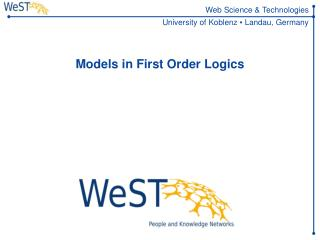DownloadDownload PresentationModels in First Order Logics

# Models in First Order Logics

Télécharger la présentation## Models in First Order Logics

- - - - - - - - - - - - - - - - - - - - - - - - - - - E N D - - - - - - - - - - - - - - - - - - - - - - - - - - -
##### Presentation Transcript

1. Models in First Order Logics

2. Overview • First-order logic. Syntax and semantics. • Herbrand interpretations; • Clauses and goals; • Datalog.

3. First-order signature • First-order signature § consists of • con — the set of constants of §; • fun — the set of function symbols of §; • rel — the set of relation symbols of §.

4. Terms Term of § with variables in X: 1. Constant c 2 con; 2. Variable v 2 X; 3. If f 2 fun is a function symbol of arity n and t1, … , tn are terms, then f(t1 , …, tn) is a term. A term is ground if it has no variables var(t) — the set of variables of t

5. Abstract and concrete notation Abstract notation: • a, b, c, d, e for constants; • x, y, z, u, v, w for variables; • f, g, h for function symbols; • p, q for relation symbols, Example: f(x, g(y)). Concrete notation: teletype font for everything. Variable names start with upper-case letters. Example: likes(john, Anybody).

6. Formulas Atomic formulas, or atoms p(t1, …, tn). (A1Æ … Æ An) and (A1Ç … Ç An) (A ! B) and (A \$ B) :A 8v A and 9vA

7. Substitutions Substitution µ : is any mapping from the set V of variables to the set of terms such that there is only a finite number of variables v 2 V with µ(v) v. Domain dom(µ), range ran(µ) and variable range vran(µ): dom(µ) = {v | v µ(v)}, ran(µ) = { t | 9 v 2 dom(µ)(µ(v) = t)}, vran(µ) = var(ran(µ)). Notation: { x1 t1, … , xn tn } empty substitution {}

8. Application of substitution Application of a substitution µto a term t: • xµ = µ(x) • cµ = c • f(t1, … , tn)µ = f(t1µ, … , tnµ)

9. Herbrand interpretation • A Herbrand interpretation of a signature § is any set of ground atoms of this signature.

10. Truth in Herbrand Interpretations 1. If A is atomic, then I ² A if A 2 I 2. I ² B1Æ … Æ Bn if I ² Bi for all i 3. I ² B1Ç … Ç Bn if I ² Bi for some i 4. I ² B1! B2 if either I ² B2 or I ² B1 5. I ²: B if I ² B 6. I ²8 xB if I ² B{x  t} for all ground terms t of the signature § 7. I ²9xB if I ² B{x  t} for some ground term t of the signature §

11. Tautology and Inconsistent A formula F is a tautology (is valid), if I ² F for every (Herbrand) interpretation I A formula F is inconsistent, if I ² F for every (Herbrand) interpretation I.

12. Types of Formulas All formulas Tautologies / Valid Neither valid nor inconsistent Inconsistent Invalid formulas

13. Logical Implication A (set of) formula(s) F logically implies G (we write F ² G), iff every (Herbrand) interpretation I that fulfills F (I ² F) also fulfills G (I ² G). ÆF ² G is true iff for every (Herbrand) interpretation I: I ² (ÆF ! G)

14. Literals Literal is either an atom or the negation :A of an atom A. Positive literal: atom Negative literal: negation of an atom Complementary literals: A and :A Notation: L

15. Clause Clause: (or normal clause) formula L1Æ … Æ Ln! A, where n ¸ 0, each Li is a literal and A is an atom. Notation: A :- L1Æ … Æ Ln or A :- L1, … , L Head: the atom A. Body: The conjunction L1Æ … Æ Ln Definite clause: all Li are positive Fact: clause with empty body

16. Syntactic Classification

17. Goal • Goal (also normal goal) is any conjunction of literals L1Æ … ÆLn • Definite goal: all Li are positive • Empty goal □: when n = 0

18. A new language Excercise: • Syntax & Semantics for Monadic Fuzzy Logics • Monadic: only unary predicates • Fuzzy: • Truth values in [0,1] • operators for truth values • T-norm: >min(a,b)=min{a,b} • T-conorm: ?min(a,b)=max{a,b} • Also: >prod(a,b)=a¢b, ?sum(a,b)=a+b-a¢b,… • Duality for N(x)=1-x